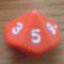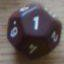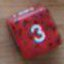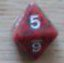3 different Dice Open ended?

## 3 different Dice Open ended?

 Thu Jul 14 21:02:45 2016   by   olytron I am experimenting with a variant rule for vehicle combat in Savage Worlds. I am adding a third open ended dice and would like to calculate the probabilities. Is this possible? I've figured out the algorithm for three d6s or if two of the three dice match like d4,d4,d6 or d8,d8,d6. But how could I calculate probabilities for a dice pool of d4,d8,d6?This is a great tool! Thanks! Thu Jul 14 21:39:52 2016   by   olytron Think I got it. I was using z as a variable and getting an error, once I used an alternate variable it was working. d4,d8,d6 Savage Worlds Stylemax { sum (accumulate x:=d6 while x=6),sum (accumulate t:=d8 while t=8) ,      sum (accumulate y:=d4 while y=4) }